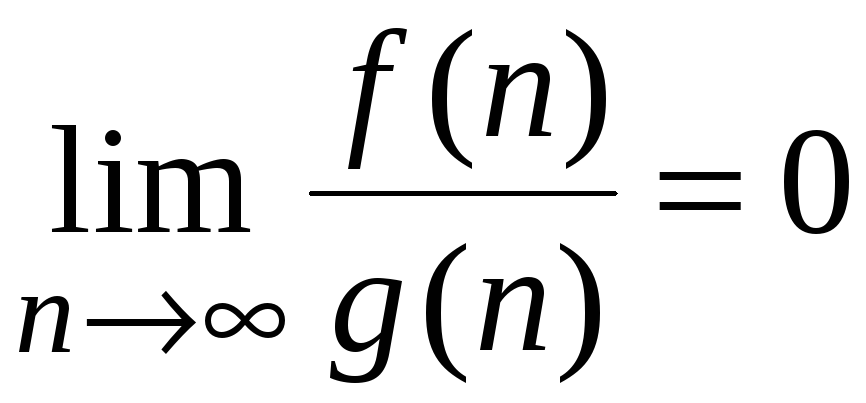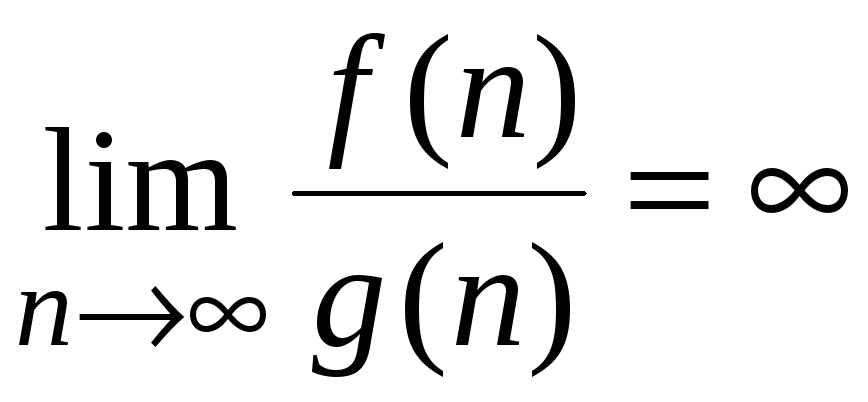# Chapter 1 Introduction Algorithms and problems An algorithm

 Page 1/3 Date 28.05.2018 Size 428.46 Kb. #51066
1   2   3

SHEN’s CLASS NOTES-F06

Chapter 1 Introduction

1. Algorithms and problems

An algorithm

is a well-defined computational procedure to solve a computational problem (or problem for short).

An algorithm must be correct. A good algorithm must be readable, time-efficient, space- efficient.
A problem

defines the requirements that valid output values must satisfy for a given set of input values.

Usually, we are only interested in those problems that allow infinitely many different sets and sizes of input values.

For example, the sorting problem takes n numbers as input set and requires the n numbers be arranged in descending or ascending order as the valid output, where the n can be any positive integer.

For a particular set of input values, the problem is referred as an instance of the problem. Therefore, a problem must contain infinitely many instances.
Solving a problem

is to find a valid output for any given input, which is done by an algorithm.

1. Relationship between an algorithm and a data structure

A data structure

is a way to store and organize data in order to facilitate accesses and modifications.

An efficient algorithm relies upon efficient data structure. No single data structure works well for all purposes. It is important to get familiar with commonly used data structures that will be overviewed in Chapters 10-14 of the textbook.

1. Relationship between an algorithm and a program

A program

is a piece of code written in a particular language such as Pascal, C, C++, Java, or even some assembly language.

Therefore, programs are language dependent or even machine dependent. A program can often be viewed as a detailed implementation of an algorithm with some limitations such as memory capacity, the largest number allowed, the largest size of an array, etc.

An algorithm is of more abstract notion. It is language independent, machine independent. An algorithm is well defined as far as its steps can clearly implemented by any known language.

Algorithms and programs are very closed related. We often use a known real language such as Pascal, C, C++ to describe an algorithm. Then, the algorithm looks like a program. However, in such a program, the grammar of the language will not be strictly followed and some notations may be changed to make the algorithm more readable. Such a language is called a pseudo language.
Example 1.
Problem: sorting n numbers

Input: A, A, …, A[n]

Output: arrange the n numbers such that

A  A  … A[n].

Algorithm Selection Sort (A[1..n]);

1. for (i = 1, in, i++)

2. do { key = i

3. for (j = i, jn, j++)

4. do if A[j] < A[key]

5. then keyj

6. A[i]  A[key]

7. End

We could call the language used in example 1 pseudo C++. However, this is not important to identify which language it looks like. We accept the presentation of an algorithm as far as its steps are clearly implementable. Example 2 shows another way to present the same sorting algorithm of Example 1.

Example 2.

Algorithm Selection Sort (A[1..n]);

1. for (i = 1, in, i++)

2. do { find j such that

3. A[j]= Min{A[i], A[i+1], …, A[n]};

4. A[i]  A[j]

5. }

6. End

By allowing this kind of abstraction, we can concentrate on design methodology and pay less effort to the implementation details.

If an algorithm is implemented by a program with a particular language, you may call such program an algorithm also. However, sometimes, a piece of program solves only a particular instance of a problem or several instances of a problem. For example, a program may be written to compute the average temperature from the data collected from fixed 10 locations. We can hardly call them an algorithm.

1. Performance of an algorithm

An algorithm must be correct, highly readable, and efficient. By efficient, we mean that the algorithm must use as little memory space as possible and use as little time as possible. The memory space needed and the time used are called space complexity and time complexity, respectively. Normally, the two complexities are related to the input size. For example, sorting 10,000 numbers will take much larger space and longer time than that of sorting 100 numbers. Also, large numbers may take longer time to process than small numbers. Therefore, the space or time complexity is represented by a function that reflects the relationship between the space or time required by the algorithm and the input size. We need some assumptions on the input size.

#### The models of input size

There are two commonly used models.

1. Use the number of numbers or number of set elements as the input size. For example, if we are given n numbers to sort, then the input size is n. In this model, we assume one memory cell will hold a number no matter how big the number is. Moreover, we assume that an operation between any two numbers takes an equal constant time. For example, (5 + 7) will takes the same amount of time as that for (10225560 + 45787899909).

1. The number of bits (or digits) that are used for encoding the set of input values. This model uses the number of bits fed into a computer as the input size. This model is useful when a number is so large such that the assumption that any operation takes a constant time makes no sense. Moreover, the input may contains only one number or few numbers. For example, if we want to determine if a given number x is a prime number, the input size should not be considered to be one. We need infinitely many different input sizes! For the prime number problem, we use lgx to be the input size because lgx bits are used to encode the number x.

In most cases, we use model (1).

#### How to represent the time complexity and space complexity

The time complexity of an algorithm is represented by a function from the input size to the time required by the algorithm to produce corresponding result.

The space complexity is represented by a function from the input size to the total number of different memory cells required by the algorithm to produce the result. This book will focus on the time complexity.
Because different machines have different speed, the same algorithm may need different time to finish on different machines. In order to fairly judge the time efficiency of an algorithm, we count the number of basic operations executed by the algorithm and use this number to represent the time complexity of a given algorithm. This number will not change from one machine to another. Moreover, we also ignore the effect caused by different compilers. We count the number of operations in the pseudo source code.
Because counting exactly the number of operations is difficult and not necessary, we usually pay more attention to the asymptotic behavior of the time complexity when the input size n goes to infinity. In other words, we pay more attention to the order or rate the complexity function grows when n goes to infinity.
For example, given two functions, f(n) = 2n and g(n) = 9n, we would consider them to be in the same order.
However, if f(n) = 200n and g(n) = n2, then g(n) grows much faster than f(n) although f(n) may be larger than g(n) when n is not very large. We would say that f(n) has smaller and better time complexity.
We wish to design an algorithm whose complexity has lower order.

#### The Growth of Functions (O, ,  notations)

We introduce some notations used to compare growth rate between two complexity functions. We assume all complexity functions have positive values.

Definition 1 Let f(n) and g(n) be two functions from the set of non-negative integers to the set of real numbers. We say that f(n) is O(g(n)) (often written as f(n) = O(g(n)) if there are positive constant c and n0 such that

f(n) ≤ cg(n)

whenever n > n0.

Example 3.
n3 + 2n +5 = O(n3) (c = 10, n0 = 1),

nlgn = O(n2) (c = 1, n0 = 1).

Definition 2 Let f(n) and g(n) be two functions from the set of non-negative integers to the set of real numbers. We say that f(n) is (g(n)) (often written as f(n) = (g(n)) if there are positive constant c and n0 such that

f(n) ≥ cg(n)

whenever n > n0.

Example 4.
n3 + 2n +5 =  (n3) (c = 1, n0 = 1),

n2 =  (nlgn) (c = 1, n0 = 1),

.

Definition 3 Let f(n) and g(n) be two functions from the set of non-negative integers to the set of real numbers. We say that f(n) is (g(n)) (often written as f(n) = (g(n)) if and only if f(n) = O(g(n) and f(n) = (g(n).

Example 5.
n3 + 2n +5 =  (n3).
Definition 4 Let f(n) and g(n) be two functions from the set of non-negative integers to the set of real numbers. We say that f(n) is (g(n)) (often written as f(n) = (g(n)) if for any positive number c > 0, there exists a constant n0 such that

f(n) ≤ cg(n) whenever n > n0.
Obviously, f(n) = (g(n)) means.
Example 6.

200n = o(nlgn)

Definition 5 Let f(n) and g(n) be two functions from the set of non-negative integers to the set of real numbers. We say that f(n) is (g(n)) (often written as f(n) = (g(n)) if for any positive number c > 0, there exists a constant n0 such that

f(n) ≥ cg(n)

whenever n > n0.

Obviously, f(n) = (g(n) means.
Note.  - notation is very seldom used.
The following is a list of commonly used representative functions in the order of their growth-rates:
lglgn, lgn, n, nlglgn, nlgn, n(lgn)2, n2, n3, 2n, n!, nn.